Courses

# Test: Properties of Gases - 2

## 10 Questions MCQ Test Topicwise Question Bank for Mechanical Engineering | Test: Properties of Gases - 2

Description
This mock test of Test: Properties of Gases - 2 for Mechanical Engineering helps you for every Mechanical Engineering entrance exam. This contains 10 Multiple Choice Questions for Mechanical Engineering Test: Properties of Gases - 2 (mcq) to study with solutions a complete question bank. The solved questions answers in this Test: Properties of Gases - 2 quiz give you a good mix of easy questions and tough questions. Mechanical Engineering students definitely take this Test: Properties of Gases - 2 exercise for a better result in the exam. You can find other Test: Properties of Gases - 2 extra questions, long questions & short questions for Mechanical Engineering on EduRev as well by searching above.
QUESTION: 1

### The equation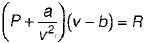is known as

Solution:

The equation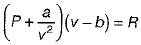is know n as Van der Waal’s equation
where,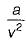= Forces of cohesion
b = Co-volume

QUESTION: 2

### Which of the following conditions are favourable for liquefaction of a gas?

Solution:

As Tr < 1, the gas becomes liquified and during phase transition isotherms are vertical. The minima of all these isotherms lie in the liquid zone

QUESTION: 3

### If value of n is zero in a polytropic process PVn = C, then the process is known as constant

Solution:

If n = 0, process is known as isobaric process

QUESTION: 4

For an ideal gas the value of Joule Thomson coefficient is

Solution:

When an ideal gas is made to undergo throttling process, there is no change in temperature hence
μ = 0

QUESTION: 5

Change in internal energy of small temperature change ΔT for ideal gas is expressed by the relation (for unit mass)

Solution:
QUESTION: 6

Change in enthalpy for small temperature change ΔT for ideal gas for unit mass is expressed by the relation

Solution:
QUESTION: 7

In Van der Waal’s gas equation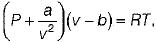The unit of b is

Solution:
QUESTION: 8

Ratio of specific heat for an ideal gas is given by

Solution: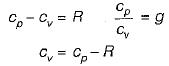Hence,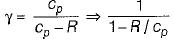QUESTION: 9

The relation pvγ= constant, where γ is the ratio of the specific heats of ideal gas is applicable to

Solution:
QUESTION: 10

When a gas is to be stored, the type of compression that would be ideal is

Solution:

For isothermal compression, work of compression is minimum hence isothermal compression is a ideal compression process.